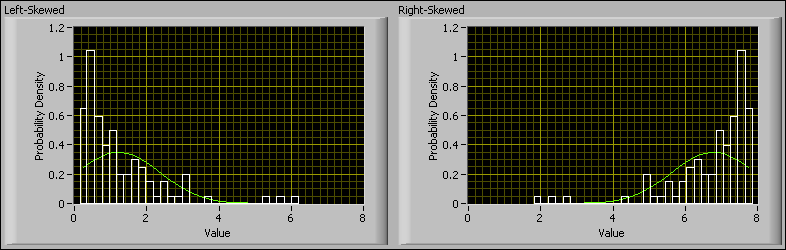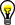# Understanding Basic Statistical Values (Advanced Signal Processing Toolkit)

LabVIEW 2014 Advanced Signal Processing Toolkit Help

Edition Date: June 2014

Part Number: 372656C-01

»View Product InfoDownload Help (Windows Only)

The basic statistical analysis methods enable you to compute statistical values for a univariate or multivariate time series, including mean, variance, skewness, kurtosis, confidence limits, stationarity, and whiteness.Note  LabVIEW Full and Professional Development Systems also contain Probability and Statistics VIs that implement similar statistical analysis methods. The Probability and Statistics VIs support univariate signals only. The Statistical Analysis VIs in the LabVIEW Time Series Analysis Tools provide more functionality and more options, such as geometric average and trimmed average, than the Probability and Statistics VIs in LabVIEW.

## Understanding the Mean and Variance Values

Mean and variance are fundamental statistical attributes of a time series. The arithmetic mean of a time series is the average or expected value of that time series. In some cases, the mean value of a time series can be the operating point or working point of a physical system that generates the time series. In general, you must subtract the value of the operating point from a time series before building predictive behavioral models for that time series. Median and trimmed mean are more robust than arithmetic mean and geometric mean if the raw data does not contain significant outliers. Use the TSA Mean VI to obtain the median or different mean values of a time series.

The variance of a time series measures the dispersion of the time series data samples around the mean value. Use the TSA Deviation and Variance VI to obtain the variance value of a time series.

## Understanding the Skewness and Kurtosis Values

The skewness and kurtosis are higher-order statistical attributes of a time series. Skewness indicates the symmetry of the probability density function (PDF) of the amplitude of a time series. A time series with an equal number of large and small amplitude values has a skewness of zero. A time series with many small values and few large values is positively skewed (right tail), and the skewness value is positive. A time series with many large values and few small values is negatively skewed (left tail), and the skewness value is negative.

The following figure illustrates two time-series PDFs with opposite skewness. Each bin in these histograms indicates the probability that any particular sample from the corresponding time series has a value within the range of that bin.The Left-Skewed graph shows a time series that is positively skewed. The Right-Skewed graph shows a time series that is negatively skewed. The green lines in these plots are the best-fit normal distributions for the given distributions.

Kurtosis measures the peakedness of the PDF of a time series. A kurtosis value close to three indicates a Gaussian-like peakedness. PDFs with relatively sharp peaks have kurtosis greater than three. PDFs with relatively flat peaks have kurtosis less than three. The following figure illustrates two time series with different peakedness.The histogram on the left shows a time series with a peaked distribution (kurtosis = 10.9817). The histogram on the right shows a time series with a flat distribution (kurtosis = 1.90294). The green lines in these plots are the Gaussian PDFs with the kurtosis equal to three.

Changes in skewness and kurtosis of a time series indicate systematic changes in the associated physical systems. Use the TSA Skewness and Kurtosis VI to obtain the skewness and kurtosis values of a time series.

## Understanding the Confidence Limits

Confidence limits are user-specified probability bounds for the range of statistical parameters. Confidence limits are useful because the estimated values of statistical parameters, such as mean and variance, typically differ for different sections of a time series. Instead of calculating one value for mean and standard deviation for an entire time series, you can generate a range of values for the mean and standard deviation over that time series by specifying confidence limits.

The estimation interval for a given set of confidence limits indicates how much the calculated mean and standard deviation values can vary across the time series. A narrow estimation interval for a parameter implies that the parameter varies little across the time series.

Use the TSA Confidence Limits VI to perform interval estimations on the statistical parameters of a time series.

## Testing the Normal Distribution

A time series whose PDF approximates a Gaussian distribution are called normally distributed. Many statistical analysis methods work well only for time series that are normally distributed. Furthermore, some modeling methods assume that the measurement noise in a time series is Gaussian white noise. For these methods, you can measure how well the histogram of a time series or measurement noise approximates a normal distribution and determine appropriate statistical analysis and modeling methods.

A normal distribution test plots the calculated distribution for a time series and its best-fit normal distribution together. If the two distributions show a close correlation, the calculated distribution for the time series is normally distributed.

Use the TSA Normal Distribution Test VI to test how normally a time series is distributed.

## Checking the Stationarity and Whiteness

Time series analysis methods can produce satisfactory analysis results for stationary time series but might not provide satisfactory analysis results for nonstationary signals. You can run a stationarity test to check if a time series is stationary.

For nonstationary signals, use other analysis methods such as time-frequency analysis and wavelet analysis.Tip  You potentially can transform a nonstationary time series into a stationary time series by removing any trend or slow variation from the time series. You also can slice the time series into approximately stationary sections and separately analyze each section.

Whiteness tests examine the spectral flatness of a time series and indirectly indicate statistical independence of a time series data samples. You also can use whiteness tests to analyze the fitness of a particular time-series model created with some modeling methods. For those methods, if the residual estimation noise for a particular model is white with a specified confidence level, the estimated model is a good fit.

Use the TSA Stationarity Test VI and the TSA Whiteness Test VI to check the stationarity and whiteness of a time series.